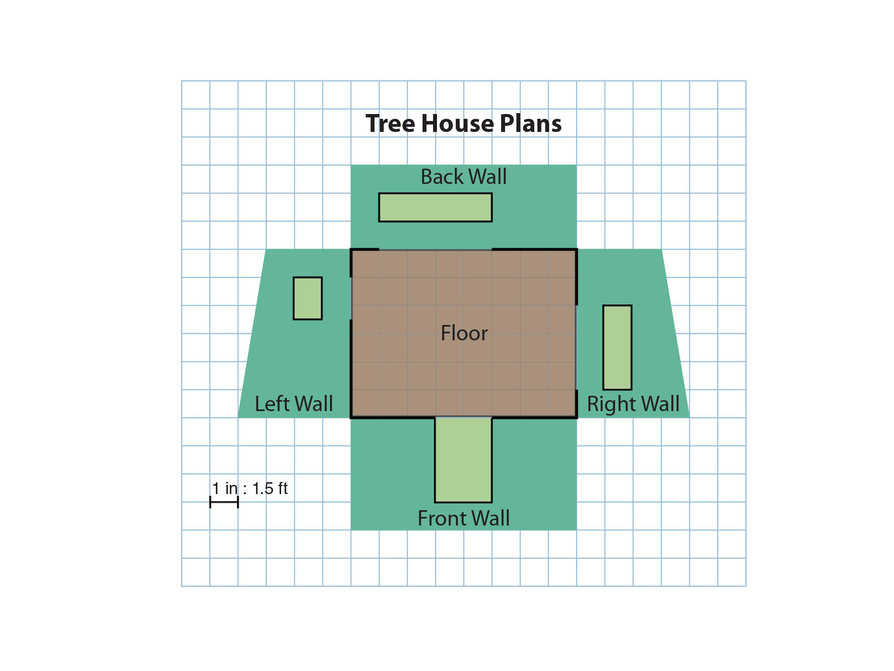Subject:
Geometry
Material Type:
Lesson Plan
Level:
Middle School
7
Provider:
Pearson
Tags:
7th Grade Mathematics, Circles, Problems, Ratio, Scale Drawing
Language:
English
Media Formats:
Text/HTML

# Gallery Problems Exercise# Gallery Overview

Allow students who have a clear understanding of the content thus far in the unit to work on Gallery problems of their choosing. You can then use this time to provide additional help to students who need review of the unit’s concepts or to assist students who may have fallen behind on work.

# Problem Descriptions

Sprinklers
Explore different sprinkler layouts, looking at circular areas (and partial circles) to decide which will be best to water a lawn.

Leaning Tower
Choose a scale and use a ruler and protractor to make a simple scale drawing.

Pizza Doubler
If you could choose between doubling the fraction of the pizza that a slice is, or doubling the radius, which option would give you more pizza? In this problem you will investigate which choice gives a bigger slice.

Area and Scale
When a figure is redrawn at a larger scale the side lengths increase by the factor of the scale (if the scale doubles the size, the side lengths double also). But, does the area increase the same way? Explore a dynamic sketch and see how area changes when the scale changes.

Tree House 1
Given plans for a tree house, redraw the plans at a different scale.

# Sprinklers

1. Each set of sprinklers will water an equal area of lawn. Starting with the sprinkler that covers a single whole circle, and calling that 1 circle area, the other areas can be compared to it.

The sprinklers with 2 half circles will water 1 circle area $\left(\frac{1}{2}+\frac{1}{2}=1\right)$.

The sprinklers that cover 4 quarter-circle areas will also cover 1 circle area $\left(\frac{1}{4}+\frac{1}{4}+\frac{1}{4}+\frac{1}{4}=1\right)$.

For the layout with 4 circle sprinklers, each circle has a radius that is half of the 1 circle area, so each circle’s area will be $\frac{1}{4}$ of the 1 circle area $\left[{\left(\frac{1}{2}\right)}^{2}=\frac{1}{4}\right]$. Since there are 4 circles, they will make 1 whole circle area.

Likewise, in the layout with 16 circle sprinklers, each radius is $\frac{1}{4}$ of the radius of the 1 circle, so it will have $\frac{1}{16}$ the area $\left[{\left(\frac{1}{4}\right)}^{2}=\frac{1}{16}\right]$ as the 1 circle area. However, there are 16 circles, so the total area will be 1 circle area.

Students can also solve this problem by finding the areas for each layout. As they go through this process, they will see the patterns and question why each area comes out the same. Hopefully, they will analyze further and see why the areas are the same.

# Sprinklers

Each square below represents a lawn area. The blue circles represent the area that the sprinklers would water.

1. Which set of sprinklers will water the most area of the lawn? Show how you decided which set of sprinklers to use.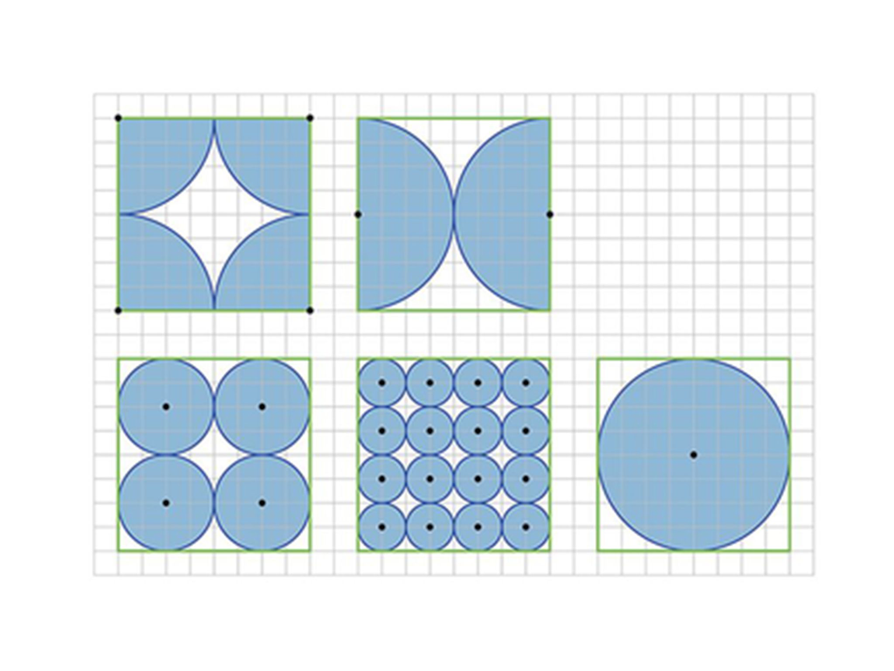# Leaning Tower

1. This task requires students to choose a scale, use a ruler to make a simple scale drawing involving perpendicular line segments, and measure an acute angle using a protractor. Students must choose a scale that is appropriate for the size of paper (or screen) that they have. If students use the centimeter grid layout, they will have to calculate what each centimeter would represent. For example, if the grid were 30 squares long, each centimeter could be 6 feet (6 • 30 = 180). Students should also realize that the larger the scale, the more difficult it will be to measure the 17 feet accurately. Conversely, they should also notice that the angle is not affected very much, so their answer for the angle will be fairly accurate.
2. Diagram: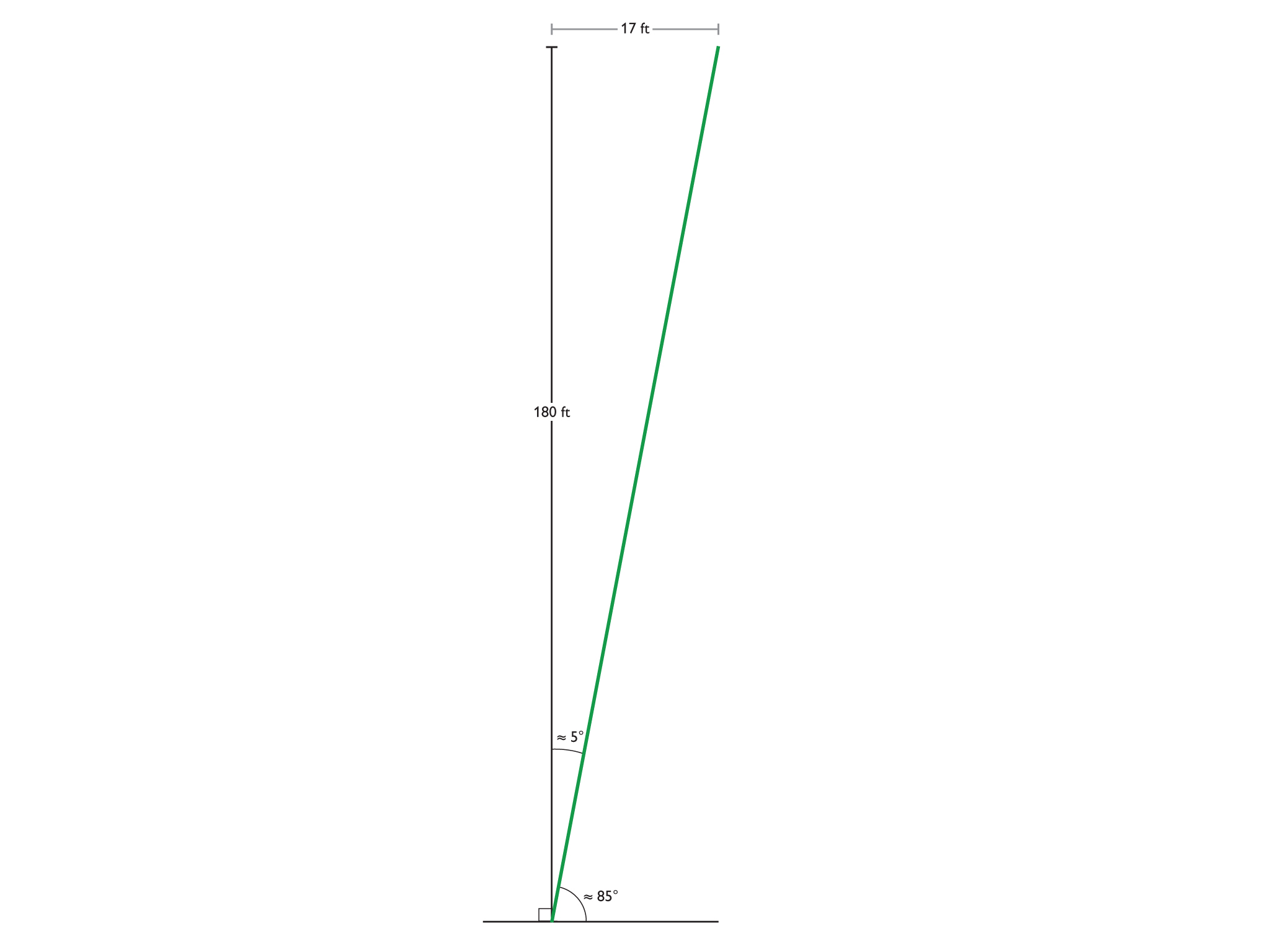3. The tower is leaning at an angle of 5°.

# Leaning Tower

Here are some facts about the Leaning Tower of Pisa, in Italy:

• The tower is 180 ft tall.
• The top of the tower is 17 ft off of vertical. That means the top is 17 ft over from where it would be if the tower were perpendicular to the ground.
1. Make a scale drawing that shows how much the tower is leaning.
• You do not need to draw the tower itself, just show a line for the tower and a line for the ground.
• Choose a scale and include it in your drawing.
2. Since the tower is leaning, it forms an angle with the ground that is less than 90 degrees. Measure this angle with a protractor. What is the measure of this angle?
3. At what angle is the tower leaning?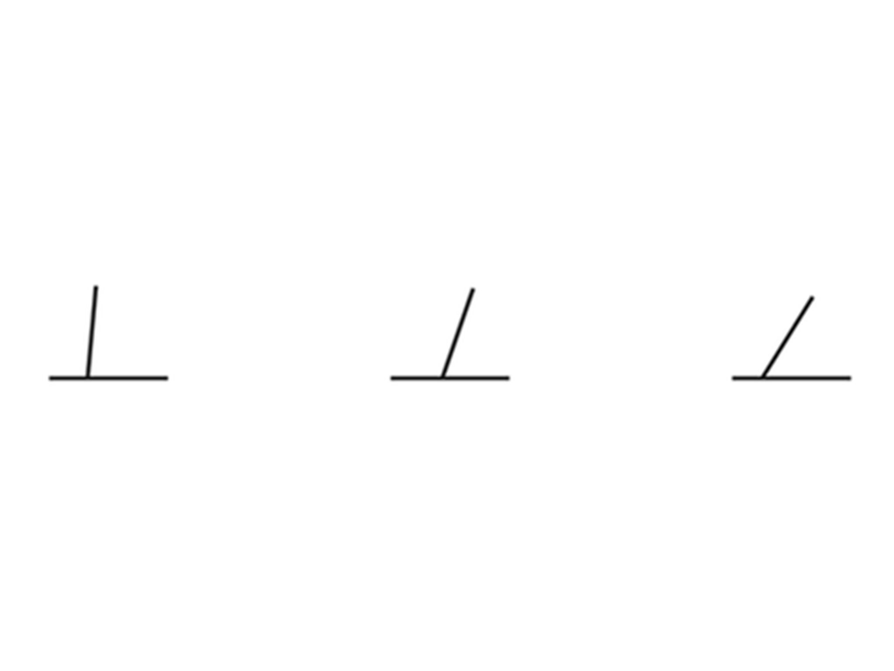# Pizza Doubler

1. Doubling the radius will give a larger slice. If the fraction of the pizza is doubled, then the area of the slice will double. However, if the radius is doubled, the area will quadruple. For example, the area of a slice from a pizza with a 10 in. radius is calculated as follows:

$A=\frac{\pi {r}^{2}}{8}$

$A\approx \frac{3.14{\left(10\text{in.}\right)}^{2}}{8}$

$A\approx \frac{314\text{}{\text{in.}}^{2}}{8}=$ 39.25 in2

If the fraction is doubled, the area will double:

39.252 • 2 = 78.52

If the radius is doubled (to $20$ in this example), the area will quadruple:

$A=\frac{\pi {r}^{2}}{8}$

$A\approx \frac{3.14{\left(20\text{in.}\right)}^{2}}{8}$

$A\approx \frac{1,256\text{in}{\text{.}}^{2}}{8}=157\text{}{\text{in.}}^{2}$ ($39.25×4=157$)

2. The coupon that doubles the radius will always be better because it will increase the area by the square of the change in the radius: 22, or 4.  Because the radius is squared in the equation, any change will be squared also. Since the radius is doubled, the area will be 4 times larger, versus only 2 times larger with the fraction-doubling coupon.

# Pizza Doubler

1. You have two pizza coupons. One states you can have a slice that is double the fraction of the whole pizza of the slice shown. The other states that you can have a slice that has twice the radius as the slice shown. Which coupon will give you more pizza? How do you know?
2. If you could use the coupon for any size slice, which coupon would you use? Why?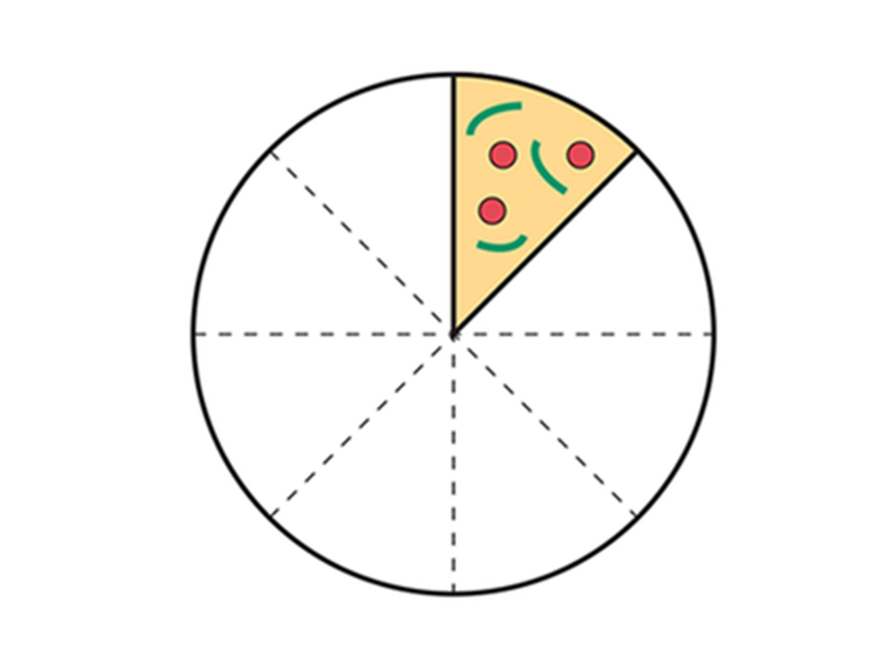# Area and Scale

1. This task gives students an opportunity to explore how area changes when scale changes. They have seen how length changes and have looked at some area examples, but they have not investigated the topic yet.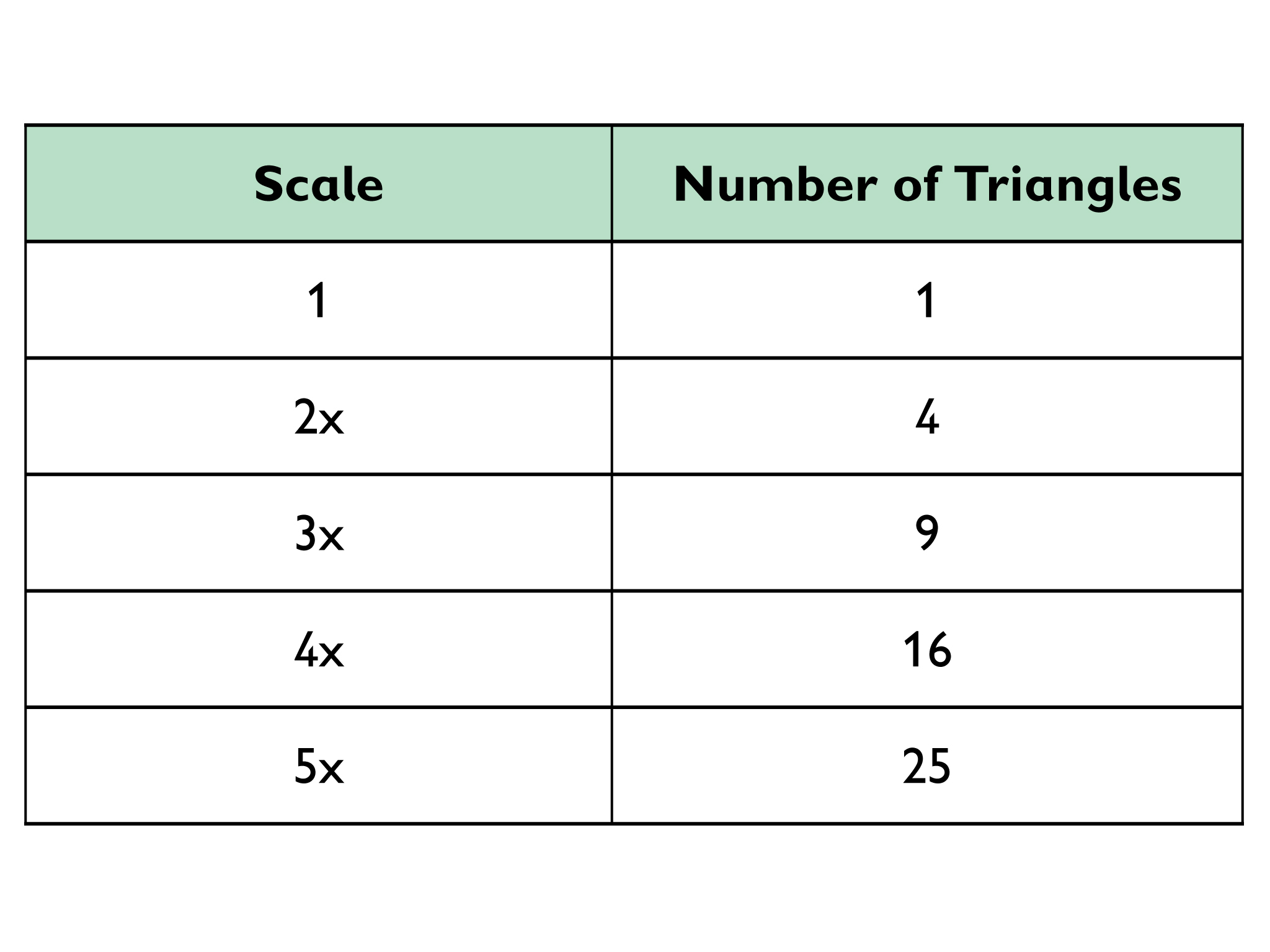2. The number of triangles is the square of the scale factor.

3. The area increases by the square of the scale factor.
4. There are 3 triangles in the smallest pentagon, 12 in the second pentagon, and 27 in the third pentagon.
5. Each triangle in the original pentagon is increasing by the square of the scale factor, so the whole pentagon is increasing the same way.

First Pentagon: 3 triangles • 12 = 3 triangles

Second Pentagon: 3 triangles • 22 = 12 triangles

Third Pentagon: $3$ triangles • 32 = 27 triangles

6. The area of any polygon will increase by the square of the scale factor because any polygon can be broken up into triangles the same way the pentagon was divided.

# Area and Scale

1. Open the Area and Scale: Triangles handout and look at the triangle with its scale increased one, two, three, four, and five times. For each Scale Level, count the number of small triangles inside and record this number in the table.
2. What do you notice about the number of triangles?
3. You can think of the area in terms of the number of triangles. How does the area change as the scale changes?
4. Open the Area and Scale: Pentagon handout. It shows a pentagon with its scale increased two times and three times. The pentagon has been divided into triangles, as have the larger pentagons. Count the number of triangles for each Scale Level, and record your answers in the table.
5. How is the area increasing?
6. How do you think the area would increase as the scale increased for any polygon?

HANDOUT: Area and Scale: Triangles

HANDOUT: Area and Scale: Pentagon

# Tree House 1

1. Answers will vary. This task gives students further practice at redrawing a scale drawing at a different scale. Students will first need to find the actual lengths so that they can be applied to a new scale. They should use their centimeter grid layout. Some students may choose to make the drawing smaller, which will be more difficult to draw accurately. A scale of 1 = 0.5 $1$ would be handy because all of the lines would fall on the grid, although there are a number of other scales that could be used.
2. Students will realize, as they read through the problem, that this question should be answered first. Floor Area: 8 in. long $\to 8$ in. • 1.5$\frac{\text{ft}}{\text{in.}}=12$ ft.

6 in. long $\to 6$ in. • 1.5 $\frac{\text{ft}}{\text{in.}}=9$ ft.

12 • 8 = 108

The floor area is 108 ft2.

# Tree House 1

The plans show the floor plan for a tree house, as well as each side. Each inch on the grid paper represents 1.5 ft in real life.

1. Redraw the plans at a different scale.
2. Find the actual area of the floor of the tree house.